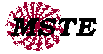# Generating Pythagorean Triples

### Description

Pythagoras was a 6th Century BC philosopher and mathematician who is famous for articulating the classic right triangle relationship:  a2+b2=cwhere a and b are the lengths of the legs and c is the length of the hypotenuse.  Sets of (a,b,c) where all three lengths are integers are called Pythagorean Triples.  In the study of the Pythagorean Theorem, students become familiar with the smaller Pythagorean Triples, such as (3,4,5) and (5,12,13).  The Java applet below generates non-trivial Pythagorean Triples of increasing value.  It can be used to locate Pythagorean Triples of significant size.

Applet Source, Written by Nicholas Exner.
Borrowed code from
triples.c by Geoffrey Coram.

### Calculator Programs for the TI-83 and TI-89, Get Graph-Link to View

Calculator Programs, Written by James P. Dildine, 1999.
Borrowed Code from All-over-the-placeSend comments to